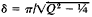# Logarithmic Decrement

## logarithmic decrement

[′läg·ə‚rith·mik ′dek·rə·mənt]
(physics)
The natural logarithm of the ratio of the amplitude of one oscillation to that of the next which has the same polarity, when no external forces are applied to maintain the oscillation.
McGraw-Hill Dictionary of Scientific & Technical Terms, 6E, Copyright © 2003 by The McGraw-Hill Companies, Inc.
The following article is from The Great Soviet Encyclopedia (1979). It might be outdated or ideologically biased.

## Logarithmic Decrement

a quantitative characteristic of the rate of damping of oscillations. The logarithmic decrement δ is equal to the natural logarithm of the ratio of two successive maximum deflections x of an oscillating quantity in the same direction: δ = log(x1/2). It is the inverse of the number of oscillations after which the amplitude attenuates by e times. For example, if δ = 0.01, then the amplitude will be reduced by e times after 100 oscillations. The logarithmic decrement characterizes the number of cycles in the course of which damping of oscillations takes place, not the time of such damping. The total damping time is determined by the ratio T /δ, where T is the oscillation period.

The usual magnitudes of the average values of the damping decrements of some systems are δ ≃ 0.1 for the acoustic oscillations of a system; δ ≃ 0.02-0.05 for an electrical circuit; δ ≃ 0.001 for a tuning fork; and δ ≃ 10i-4- 105. for a quartz plate. Hence it can be seen that, for example, a tuning fork completes about 1,000 oscillations before the amplitude of the oscillations is reduced by a factor of 3, since e = 2.718 ~ 3.

In the theory of forced oscillations the concept of the quality Q of an oscillatory system, to which the logarithmic decrement is associated by the relationship, is usually used instead of the logarithmic decrement. For high quality factors, δ =π/Q

### REFERENCE

Strelkov, S. P. Vvedenie v teoriiu kolebanii, 2nd ed. Moscow, 1964.

V. N. PARYGIN

References in periodicals archive ?
The static and dynamic moduli, the hysteresis losses and the logarithmic decrement were measured on a Yerzley mechanical oscillograph AYO-IV in accordance with ASTM D-945.
Physical properties (density and moisture content) and acoustic variables (longitudinal vibration frequency, dynamic modulus of elasticity [dMOE], and logarithmic decrement [LD]) were obtained for every piece.
The coefficients of the logarithmic decrement of damping for the obtained dependences of the oscillation amplitude of mass of the vibroprotection system with the elastic element and ECDM versus time (Fig.
Logarithmic decrement method is often used to calculate the damping ratio of structure and the equation is shown:
The temperature dependences of the logarithmic decrement [delta] (T) and the resonant frequency f(T) of the composite vibrator were measured.
The logarithmic decrement method was used to determine the damping ratio of the fruit-rachilla system in accordance with Equation 2 proposed by RAO (2008).
The logarithmic decrement of the damping frequency that characterizes muscle elasticity is calculated as decrement = ln([[alpha].sub.max]/[alpha]), where [[alpha].sub.max] is the maximal amplitude of oscillation and [alpha]T is the amplitude of the second oscillation cycle.
The logarithmic decrement method was applied to determine the modal parameters (Aristizabal et al., 2003), which is the amplitude reduction rate of a free damped vibration, defined as the natural logarithm of the ratio between any two successive amplitudes measured in the same direction (Beards, 1996).
The acceleration curve, generated via an 8 ms perturbation, yielded two outcome variables: frequency of oscillation (in hertz; an indicator of stiffness) and logarithmic decrement of damped oscillation (reflecting elasticity).
Control of the process of vibro-treatment of the construction was developed using registration of logarithmic decrement of free oscillations of constructions after impact has been applied.
where [[lambda].sub.[phi]]-the logarithmic decrement of the natural torsional oscillations; [f.sub.[phi]]-the natural frequency of torsional oscillations, Hz.

Site: Follow: Share:
Open / Close